• Print view

## Basic npc script "fails"

Description: Have any suggestions or new ideas for SMBX-38A? This is a place for you. Good suggestions may appear in future versions of SMBX-38A.
Moderators: Lx Xzit, Yoshi021, 5438A38A, Semi-moderatos, Moderators

 NESTED ERNESTTopic author, CountNESTED ERNESTTopic author, CountReputation: 71 Posts: 261 Joined: 23 Sep 2017 Location: Perú, Lima Website Post #113 Sep 2020, 2:40 This topic was very difficult to do, I really hope the developer reads it.Other possible titles:*The maximum potential of npc(x).stand*CNPC flag orientationI was programming this npc, and this idea came up:You will notice that at the end of the gif image I hit the slope and did not move forward.caminando.gif (943.51 KiB) Viewed 264 times The suggestion is to create a cnpc flag called orientation that makes the npc interpret the "gravity" differently, this value can be 0, 90, 180 and 270, the normal value is 270, this will also make the npc(x).stand and when the npc hits the "wall" it faces the other direction.(this suggestion is not that you program the rotation of images with the speed gravity)The normal value is 270(looking at a circumference) and because gravity is down normally.*cnpc orientation with value 270Programming a simple npc: SpoilerCode: Select all`with npc(sysval(param1))   .friendly=1      .ysp+=0.26   if .ysp>8 then .ysp=8      .ivala+=1   if .ivala=1 then .xsp=0.8*(1-2*.facing)      if .stand=1 then .ysp=-4end with`npc270.gif (120.44 KiB) Viewed 264 times I will highlight something:* npc(x).stand=1 works.* When npc hits the wall it flips. (Good)* There is one more feature, I show it in this image with the player(remember this as feature B) :SpoilerThe player jumps and collides with a slope below and stops him(npcs too), although this seems silly it is very important, And it is also because my npc stops at the first gif imagesaltobajom.gif (91.58 KiB) Viewed 264 times *The feature B in this case are on the slopes type bottom left and bottom right.Now we will see the others(different from 270):*cnpc orientation with value 90Programming a simple npc:SpoilerCode: Select all`with npc(sysval(param1))   .friendly=1      .ysp-=0.26   if .ysp<-8 then .ysp=-8      .ivala+=1   if .ivala=1 then .xsp=2*(1-2*.facing)      if .stand=1 then .ysp=4   end with`npc90.gif (115.1 KiB) Viewed 264 times We can say:* When npc hits the wall it flips. (Good)*The feature B in this case would be on the slopes type upper left and upper right.* For THAT gravity(because of that cnpc flag=90) you could make the npc(x).stand=1 work when colliding with its relative floor(previous image) and take advantage of your collision code;we have to write more or less something like this:(and the npc(x).stand=1 would work)SpoilerI made this code to detect collisions and slopes(npc on the player to make it faster), but we could take advantage of yoursCode: Select all`dim cal0 as doublewith npc(sysval(param1))   .friendly=1      .x=char(1).x   .y=char(1).y   .width=char(1).pwidth   .height=char(1).pheight      if v(b)<>0 then   block(v(b)).forecolor=-1   end if      .extset=0   v(a)=itrcreate(1,0,.x-2,.y-2,.width+4,.height+4)   do      v(b)=itrnext(v(a))      if v(b)=0 then exit do               select case block(v(b)).ncollision         case 4,19            if .x<=block(v(b)).x+block(v(b)).width and .y<=block(v(b)).y+block(v(b)).height then               cal0=block(v(b)).height/block(v(b)).width*(block(v(b)).x+block(v(b)).width)+block(v(b)).y               if .y>=cal0-(.x+.width)*block(v(b)).height/block(v(b)).width-.height then                  .extset=-1                  exit do               end if                                          end if                     case 5,20            if .x+.width>=block(v(b)).x and .y<=block(v(b)).y+block(v(b)).height then               cal0=-block(v(b)).height/block(v(b)).width*block(v(b)).x+block(v(b)).y               if .y>=cal0+.x*block(v(b)).height/block(v(b)).width-.height then                  .extset=-1                  exit do               end if                                          end if                     case 6,21            if .x<=block(v(b)).x+block(v(b)).width and .y+.height>=block(v(b)).y then               cal0=-block(v(b)).height/block(v(b)).width*block(v(b)).x+block(v(b)).y               if .y<=cal0+(.x+.width)*block(v(b)).height/block(v(b)).width then                  .extset=-1                  exit do               end if                                    end if                     case 7,22            if .x+.width>=block(v(b)).x and .y+.height>=block(v(b)).y then               cal0=block(v(b)).height/block(v(b)).width*(block(v(b)).x+block(v(b)).width)+block(v(b)).y               if .y<=cal0-.x*block(v(b)).height/block(v(b)).width then                  .extset=-1                  exit do               end if                                       end if                     case else            if .x+.width>=block(v(b)).x and .x<=block(v(b)).x+block(v(b)).width then               if .y+.height>=block(v(b)).y and .y<=block(v(b)).y+block(v(b)).height then                  .extset=-1                  exit do               end if                           end if      end select               loop   call berase(0,v(a))      if .extset=-1 then      block(v(b)).forecolor=rgba(255,0,0,128)   end if   end with`cnpc orientation with value 0 and 180 fails more.*cnpc orientation with value 0Programming a simple npc:SpoilerCode: Select all`with npc(sysval(param1))   .friendly=1      .xsp+=0.26   if .xsp>8 then .xsp=8         .ivala+=1   if .ivala=1 then .ysp=-2*(1-2*.facing)      if .stand=1 then .xsp=-4   end with`npc0.gif (92.54 KiB) Viewed 264 times We can say:* The wall would now be the floor, and now the npc cannot flip when it hits the wall.(fails)* Well I suppose you understand how it should work, obviously the char(x).stand=1 would be when it collides with its relative floor.* Well remember feature B would also change with each value of the cnpc flag, in this case would be on the slopes type bottom right and upper right.*cnpc orientation with value 180*Well this is similar to cnpc orientation with value 0* I suppose you understand how it should work.Its programming is:SpoilerCode: Select all`with npc(sysval(param1))   .friendly=1      .xsp-=0.26   if .xsp<-8 then .xsp=-8         .ivala+=1   if .ivala=1 then .ysp=2*(1-2*.facing)      if .stand=1 then .xsp=4   end with`npc180.gif (193.96 KiB) Viewed 264 times Attachments level.rar (178.73 KiB) Downloaded 48 times Programming NPCs in SMBX-38A is fun:That is me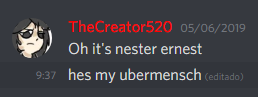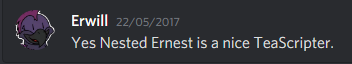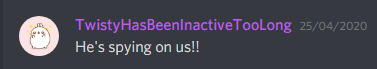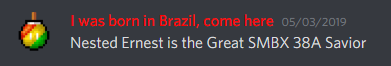ROCKMAN GIF IN SMBX 38A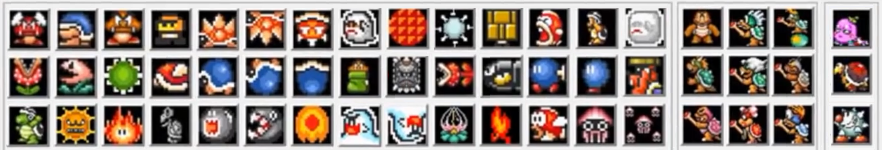MagmaBuzzPasserbyMagmaBuzzPasserbyReputation: 4
Posts: 3
Joined: 13 Aug 2020

### Nos vas a dejar sin trabajo a todos

I don't know what your doing there but you're scaring me
With that you could create as one of your Youtube Video Commenter said "The SMW Red Slope or even the Sonic Loop!"